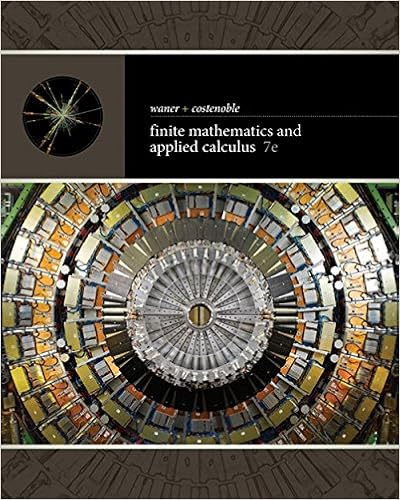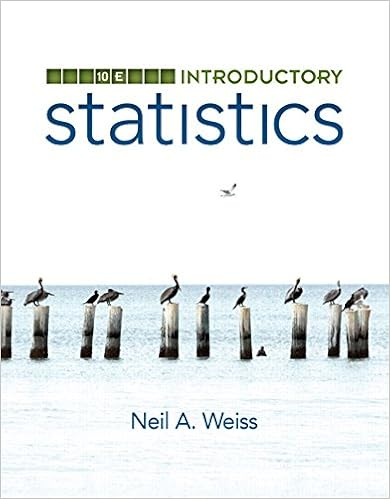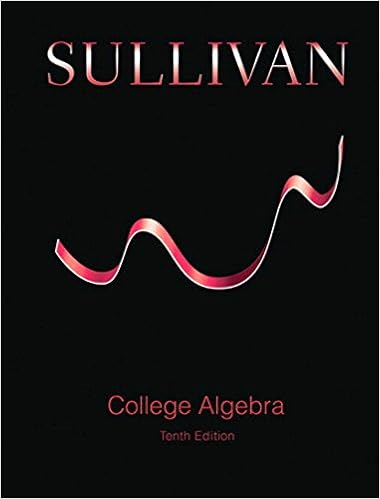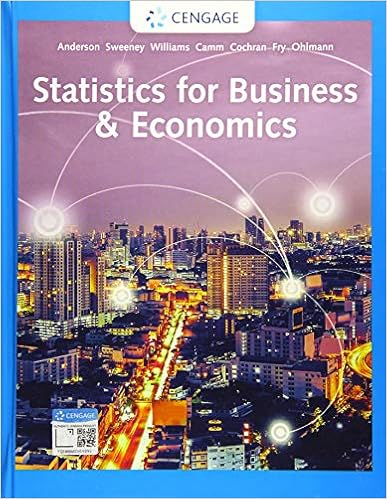# Week6Worksheet(1).pdf - MXB106 Semester 2, 2018 Week 6...

• Assignment
• 2

This preview shows page 1 - 2 out of 2 pages.

MXB106Semester 2, 2018Week 6 WorksheetWorkshopWeek 6: Eigenvalues and eigenvectorsQ5 will be done by the tutor at the end of the workshop1. If the matrixA=124-1has the eigenvalueλ= 3, show that the vectorv=11Tis the corresponding eigenvector.2. LetAandxbe as follows:A=401232104;x=121Confirm by multiplication thatxis an eigenvector ofA. What is the correspondingeigenvalue?3. LetA=13-3-37-3-66-2(a) Find the characteristic equation forA.(b) Find the eigenvalues forAand their corresponding multiplicities.(c) For each eigenvalue, find the corresponding eigenvectors.4. LetA=1-321(a) Find the characteristic equation forA.(b) Find the eigenvalues forAand their corresponding multiplicities.(c) For each eigenvalue, find the corresponding eigenvectors.5. LetA=2111211121
##### We have textbook solutions for you!The document you are viewing contains questions related to this textbook.
Chapter 5 / Exercise 77
Finite Mathematics and Applied Calculus
Costenoble/WanerExpert VerifiedBrowse all Textbook Solutions
MXB106Semester 2, 2018Week 6 WorksheetWorkshop(a) Verify that the characteristic equation ofAis (λ-4)(λ-1)2= 0.(b) Using your result from (a), find the eigenvalues ofAand their corresponding
End of preview. Want to read all 2 pages?

Course Hero member to access this document

Term
Spring
Professor
a
Tags
Eigenvalues, Eigenvalue eigenvector and eigenspace, characteristic equation, corresponding eigenvectors
##### We have textbook solutions for you!
The document you are viewing contains questions related to this textbook.The document you are viewing contains questions related to this textbook.
Chapter 5 / Exercise 77
Finite Mathematics and Applied Calculus
Costenoble/WanerExpert Verified
•••